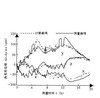β    j

k

multivariable linear regression model

• 线性回归模型建模步骤 （一元线性回归、多元线性回归
• 基于jupyter notebook的python编程—–运用sklearn库，导入文件数据模拟多元线性回归分析的目录一、运行jupyter notebook，搭建python环境1、打开Windows终端命令行，输入==jupyter notebook==，打开我们的jupyter...
• 原始数据在这里 1.观察数据 首先，用Pandas打开数据，并进行观察。 import numpy import pandas as pd import matplotlib.pyplot as plt ...我们的问题是得到一个线性的关系，对应PE是样本输出，而AT/V/
• 基于matlab的各种多元统计分析模型源代码-多元线性回归MultiLineReg.rar 本人为了获得更多资源共享的权限，只好吐血奉献自己一年来收集和改写的matlab源程序，部分为原创；里面包含有主成分分析、岭回归分析、因子...matlab
• 通过具体的案例讲解时间序列下多元线性回归在eviews里的操作
• ## 多元线性回归模型

万次阅读 多人点赞 2019-07-02 19:22:12
多元线性回归模型通常用来研究一个应变量依赖多个自变量的变化关系，如果二者的以来关系可以用线性形式来刻画，则可以建立多元线性模型来进行分析。 1.模型简介 1.1模型的结构 多元线性回归模型通常用来描述变脸y和x...
多元线性回归模型通常用来研究一个应变量依赖多个自变量的变化关系，如果二者的以来关系可以用线性形式来刻画，则可以建立多元线性模型来进行分析。 1.模型简介 1.1模型的结构 多元线性回归模型通常用来描述变量y和x之间的随机线性关系，即：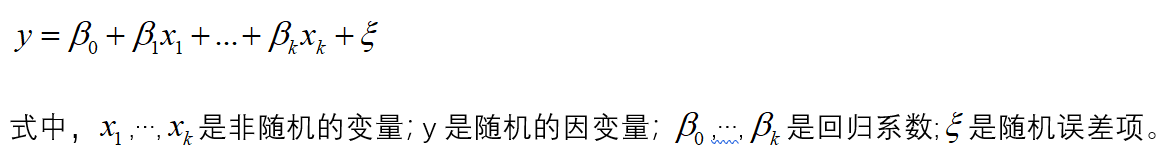如果对y和x进行了x次观测，得到n组观察值yi,x1i,…,xki(i=1,2,…,n),他们满足一下关系式：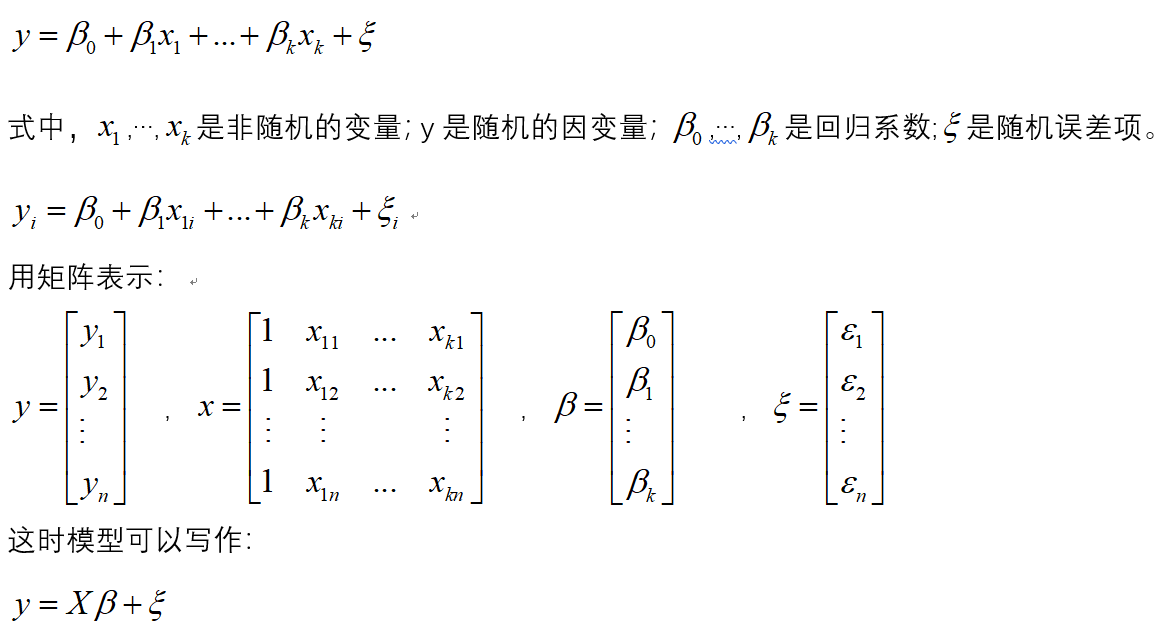1.2模型参数的检验 在正态假定下，如果X是列满秩的，则普通线性回归模型的参数最小二乘估计为：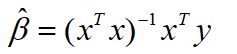于是y的估计值为：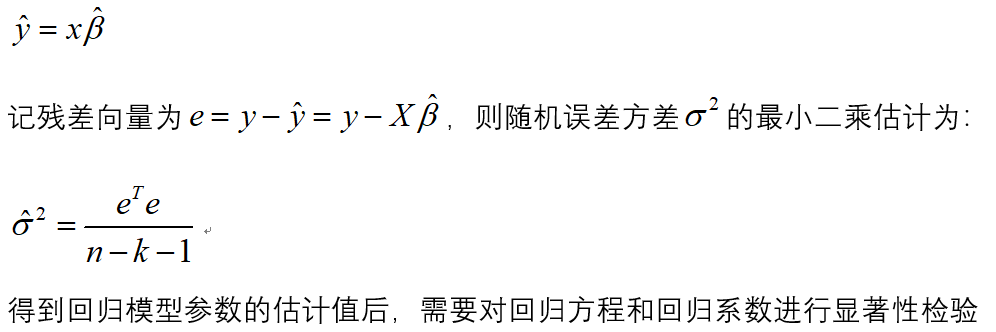（1）回归方程的显著性检验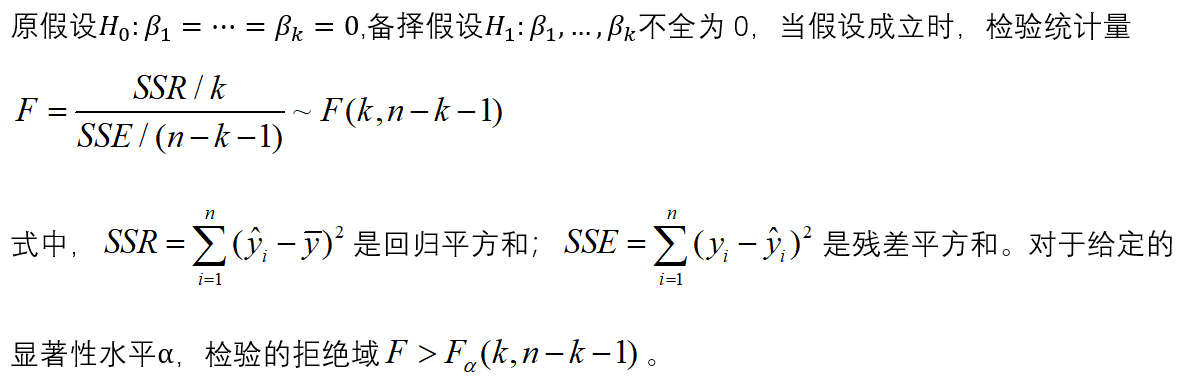（2）回归系数的显著性检验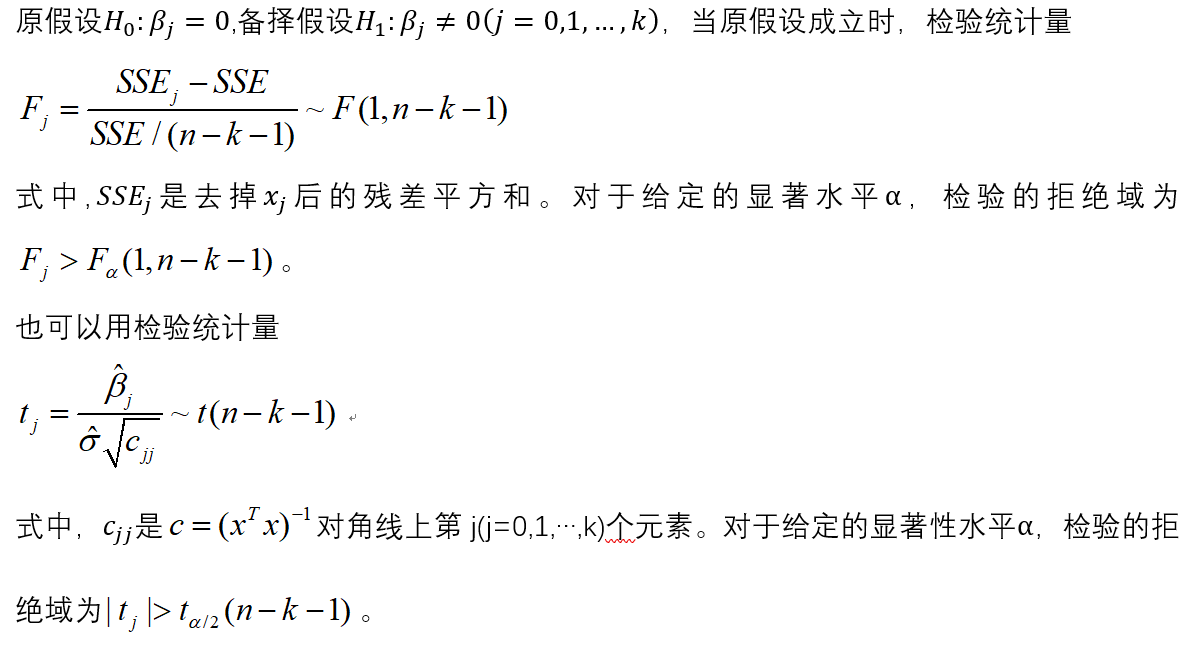2.建模步骤 （1）根据数据建立回归模型 （2）对模型进行显著性检验 （3) 对模型进行回归诊断 3.建模
library(car)
a
lm.salary=lm(锘縴~x1+x2+x3+x4,data=a)
summary(lm.salary)
#注：锘縴是y乱码之后的结果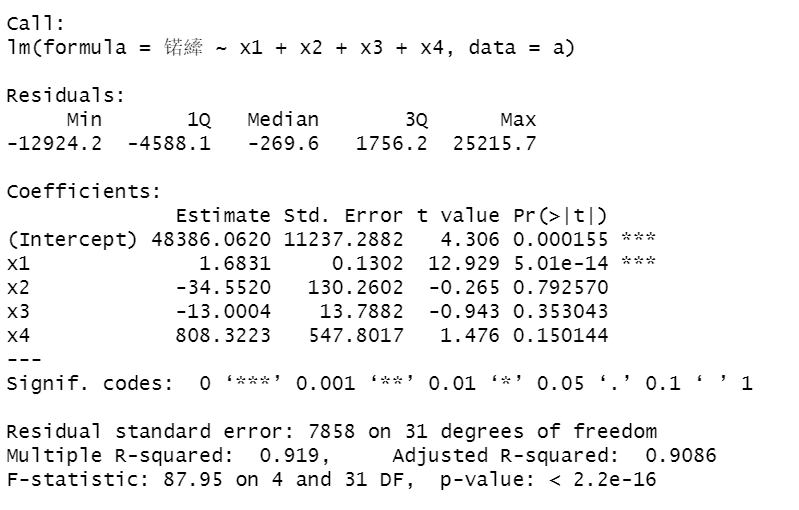发现x2,x3,x4系数不显著。 （2）对变量进行选择
lm.step=step(lm.salary,direction="both")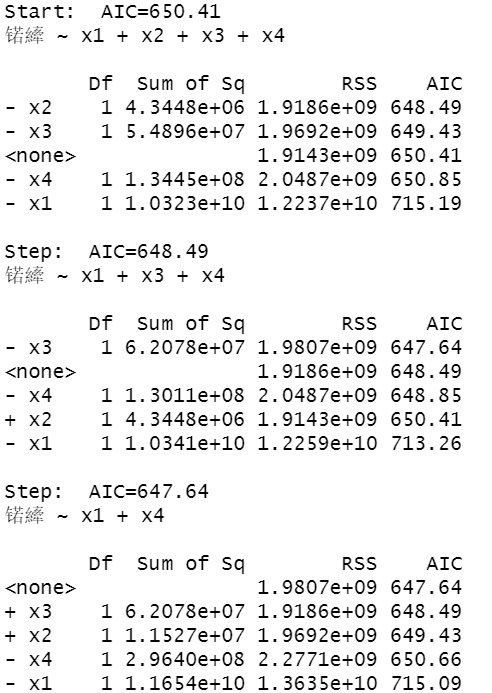如果去掉变量x2,AIC的值为648.49，如果去掉变量x3,AIC的值为650.85，如果去掉变量x1,AIC的值为715.19，所以在这里去掉x2. 进行下一轮的计算：
lm.salary=lm(锘縴~x1+x3+x4,data=a)
lm.step=step(lm.salary,direction="both")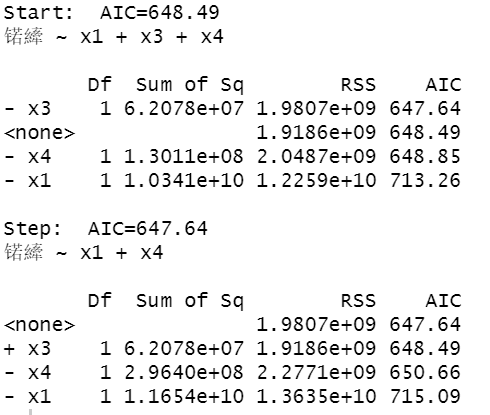发现去掉x3，AIC 的值为647.64，所以去掉x3. 单独对x1和x4，进行拟合。
lm.salary=lm(锘縴~x1+x4,data=a)
summary(lm.salary)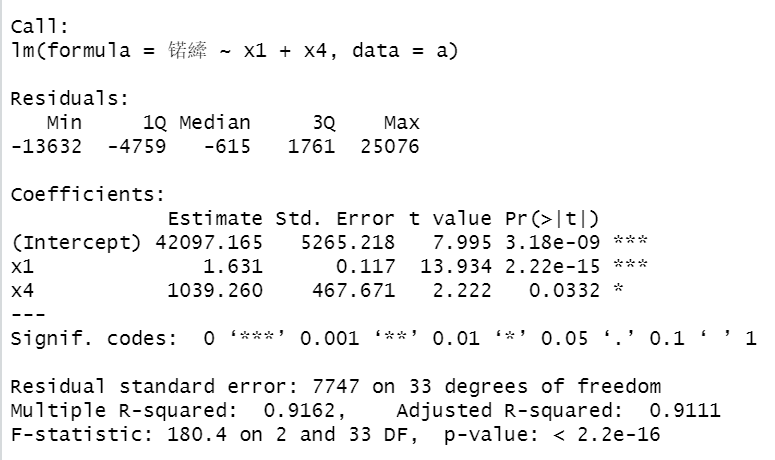可以看出F检验P值小于0.05显著，各个参数系数也是显著的。 （3）对上述回归模型进行回归残差诊断
算出模型的标准化残差
library(TSA)
y.rst=rstandard(lm.step)
y.rst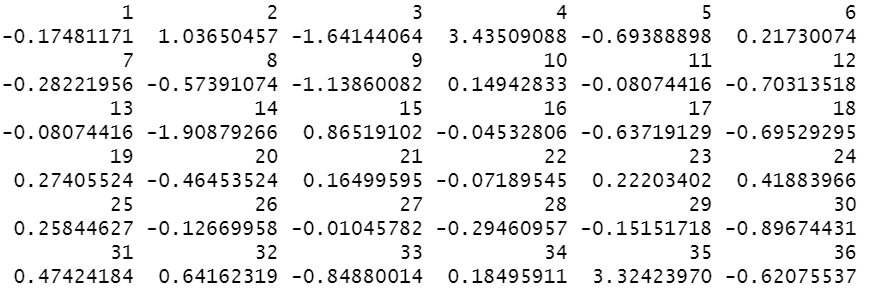画出其残差散点图：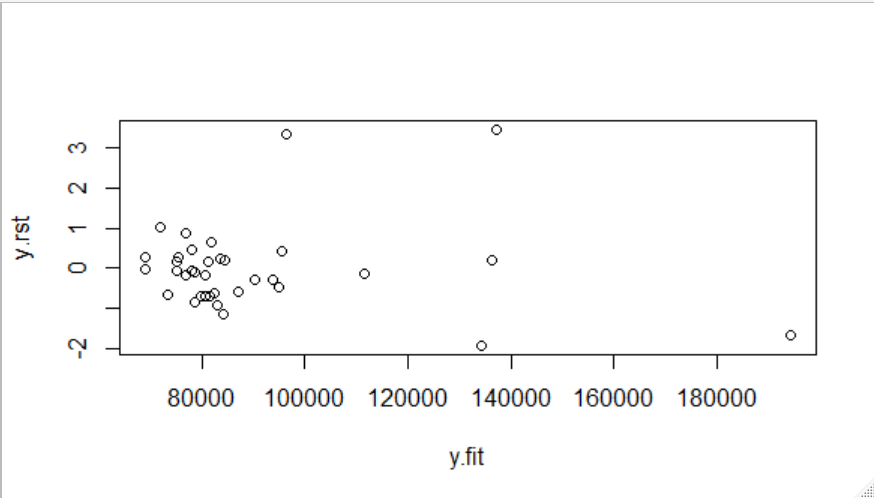很明显发现4和35号点异常，将这两个点去除。
lm.salary=lm(log(锘縴)~x1+x2+x3+x4,data=a[-c(4,35),])
lm.step=step(lm.salary,direction="both")
y.rst=rstandard(lm.step)
y.fit=predict(lm.step)
plot(y.rst~y.fit)

去除两点后的结果：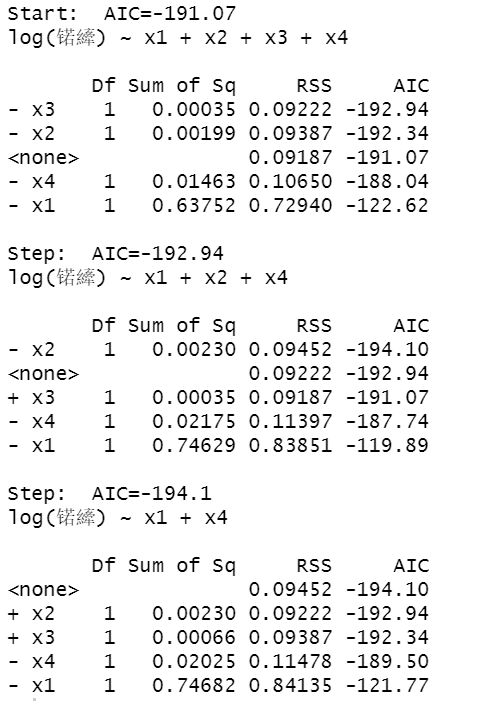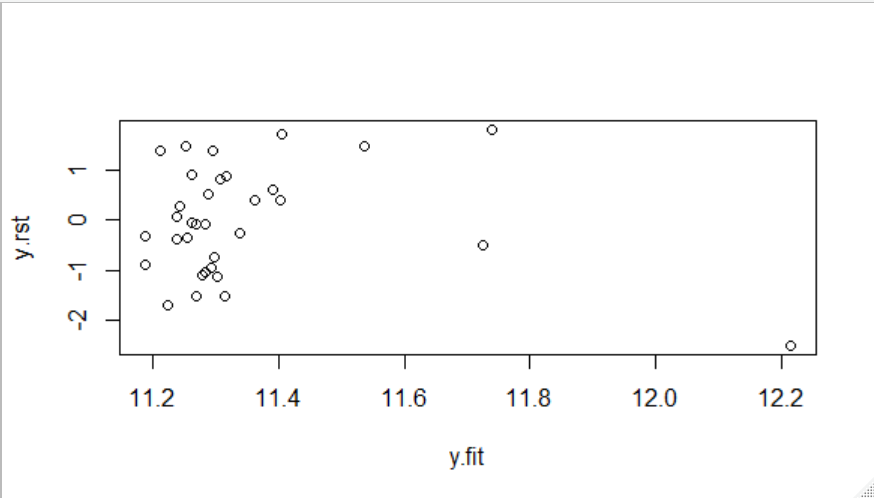绘制模型诊断图：
par(mfrow=c(2,2))
plot(lm.step)
influence.measures(lm.step)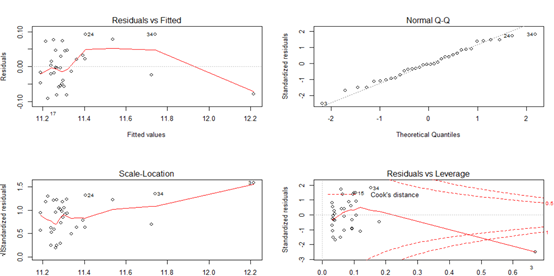残差拟合图基本上呈现随机分布模式，正态Q-Q图基本落在直线上，表明残差服从正态分布；大小-位置图和残差-杠杆图以小组的形式存在并且离中心不远。这说明3，4，35号观测值可能是异常点和强影响点。
展开全文• 使用R语言对数据分析主成分分析实现多元线性回归。包括源数据和代码。
• 终于找到一篇全面而又简洁的讲多元线性回归模型检验方法的文章，涵盖了 主要的统计检验——F检验、t检验、DW检验
• 主要用于数学建模（Matlab）的学习，下载下来换成你的数据就可以用了。
• 使用python实现多元线性回归，内容包含数据源及代码实现python
• 多元线性回归的参数估计方法，吴仕勋，赵东方，本文依据高斯—马尔可夫定理，通过对最小二乘估计方法得出的参数估计值的分析，从另外两个角度出发得出了参数估计的值与最小二乘
• 多元线性回归:在回归分析中，如果有两个或两个以上的自变量，就称为多元回归。事实上，一种现象常常是与多个因素相联系的，由多个自变量的最优组合共同来预测或估计因变量，比只用一个自变量进行预测或估计更有效，...测绘工程
• 利用多元线性回归和BP神经网络理论,分别对矿井瓦斯涌出量进行了预测,最后建立了多元线性回归与BP神经网络的组合预测模型。该模型兼顾了多元回归分析的非线性特性和神经网络的时序特性,通过具体的实例研究,对比了各种...
• 提出了一种基于遗传算法模糊多元线性回归分析的瓦斯涌出量预测模型 。 采用灰关联分析法和 ＳＰＳＳ 软件线性回归分析法确定影响瓦斯涌出量的主要因素 ； 把历史数据样本分为建模数据样本和检测数据 样本 ， 采用...
• 自行推导公式多元线性回归的编程一、导入文本店铺面积和营业额的关系图车站距离和营业额的关系图二、计算下图三、计算R² 一、导入文本 import pandas as pd import numpy as np import matplotlib.pyplot as plt ...
• 上一篇文章讲述了梯度下降法的数学思想，趁热打铁，这篇博客笔者将使用梯度下降法完成多元线性回归，话不多说，直接开始。 我们假设我们的目标函数是长这样的： import numpy as np import pandas as pd # 读入...
• 用Java完成多元线性回归相关算法编程。资源是从百度文库上下载的https://wenku.baidu.com/view/070d30eb988fcc22bcd126fff705cc1755275f61.html。
• 专门用于处理数据分析，包括多元分析线性回归分析等，简单方便，一用即知。推荐下载使用，处理数据十分方便
• 其实上一篇讨论的多元线性回归还不是很全面，而且训练和测试集都没有分，在这里继续讨论多元线性回归模型检验的相关问题。 多元线性回归的输出变量是连续变量，输入变量中如果包含离散变量需要做哑变量或One-hot编码...
https://editor.csdn.net/md/?articleId=105137945 其实上一篇讨论的多元线性回归还不是很全面，而且训练和测试集都没有分，在这里继续讨论多元线性回归模型检验的相关问题。
只要有P值的出现，样本量不超过5000，比如线性回归和逻辑回归；搞清楚算法背后的逻辑才是比较重要的。
多元线性回归需要关注一些什么点？R2和模型稳定性，也就是那些β是不是稳定的，检验模型是不是稳定需要对模型进行诊断。
多元线性回归的输出变量是连续变量，输入变量中如果包含离散变量需要做哑变量或One-hot编码，连续变量可以直接用来建模。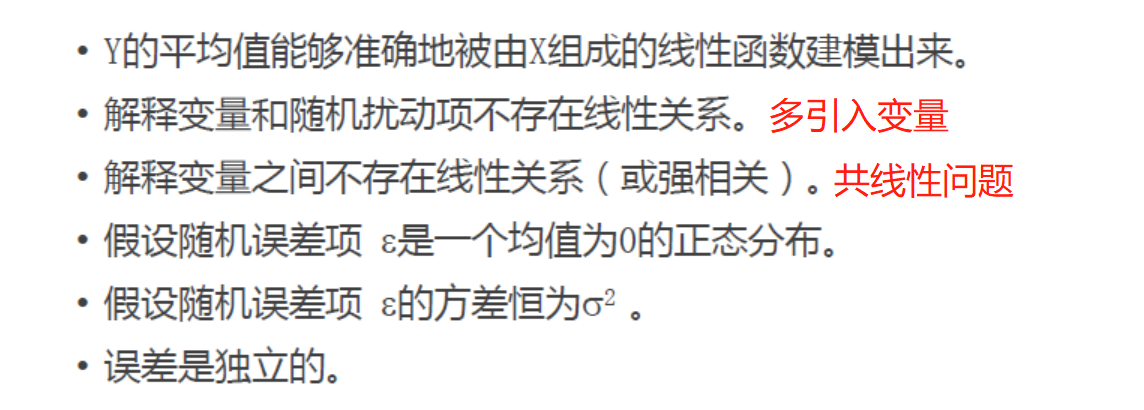多元线性回归假设解释
多元线性回归需要满足的假设其实是比较强的，但是在机器学习或者是数据挖掘领域，后3条针对误差项（其实就是残差）的假设基本上被忽略了。 第1条： 看因变量y和自变量x之间的关系，可以通过绘制散点图，确定是线性、二次函数还是指数函数关系，根据这个来建立x和y之间的关系。后面的神经网络和SVM等模型就是为了方便找到x和y之间的关系。 第2条： 解释变量和随机扰动项不存在线性关系。我们想象一下，如果他们之间存在线性关系的话，是不是会导致回归系数估计的不准确啊，举个例子解释变量y是收入，x是受教育程度，并假设回归方程是 y = 0.5x + e，设想如果扰动项里面包含父母收入，实际上父母收入会影响孩子的收入y，那么回归系数估计值0.5是不是偏高了啊。那怎么解决这个问题呢，那就多纳入一些变量来参与建模吧，这也是多元线性回归存在的必要性，同时这也引出了一个变量筛选的问题。 第3条：解释变量之间不存在线性关系（或强相关）。在建模时，我们不但需要估计回归系数的均值，还需要估计回归系数的标准差：S（β）= S（e）/ |x|，那么如果解释变量x之间存在线性关系的话，分母趋向于0了，回归系数标准差趋于无穷大，所以多重共线性问题是需要去避免的。
多元线性回归诊断方法
如果扰动项是右偏，那么残差图肯定是异方差分布，取对数即可，所以下图中假设5和假设4可以说是一致的。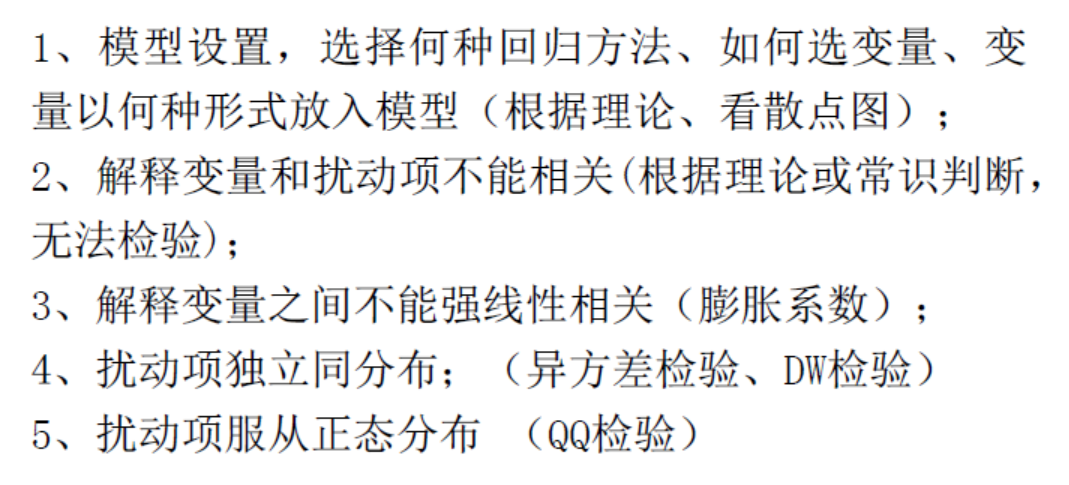多元线性回归模型的诊断
（1）残差分析：实际上当残差不包含任何信息的时候是最好的，如果还包含一些信息，需要把这个信息提取出来。残差图的纵坐标是残差，横坐标可以是各个解释变量x，实际上在做单变量分析，解释变量x被解释变量y做相关性分析的时候就知道了；比如某个解释变量x和被解释变量y都是右偏，那么残差图肯定是异方差，同时取对数重新建模；如果某个解释变量x和被解释变量y存在抛物线关系，那么加入二次项重新进行建模；自相关一般在时间序列数据中比较常见。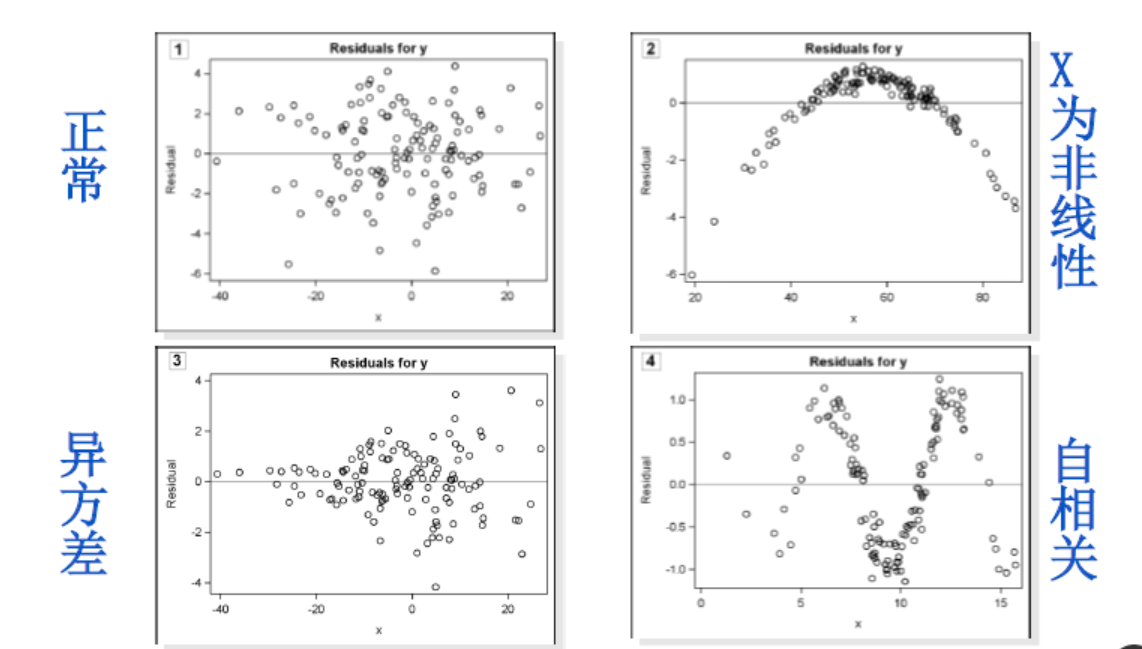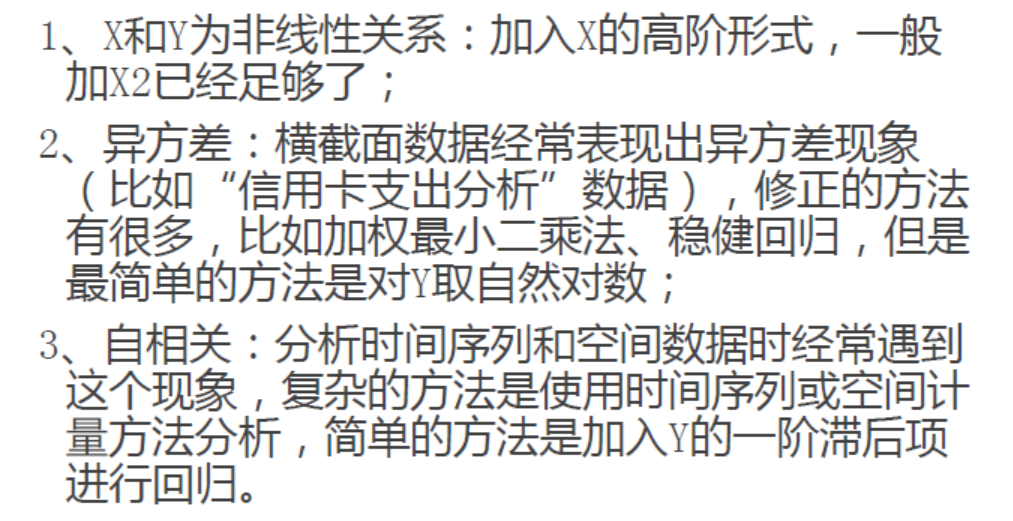（2）强影响点分析： 为什么要做强影响点分析？？？因为有了强影响点的存在之后，会把本来没有关系的数据带出关系来，而且这个关系特别不稳定。比如下图，本来数据点之间没有什么关系，但是因为强影响点的存在之后，给数据带出来了这么一个线性关系出来，但这个关系是非常不稳定的，不具有大众性。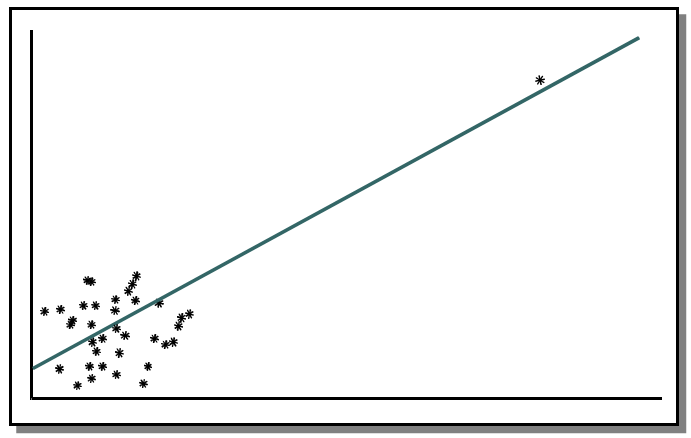怎么解决强影响点分析问题？？？学生化残差（只做一次）。
|SR| = (残差 - 残差均值) / 残差标准差。 |SR| > 2，剔除掉满足条件的记录（几百个样本） |SR| > 3，剔除掉满足条件的记录（几千个样本）
（3）共线性问题 可以参考下面的链接：https://www.sohu.com/a/326904117_100103806 共线性的判别指标：膨胀系数VIF、相关系数 共线性的解决方法：根据VIF和相关系数手动剔除变量、逐步回归法、岭回归。
展开全文多重共线性
• 一种多元线性回归模型在医学中的应用，徐云龙，李剑英，本文是关于通过化验人体内各种元素含量来判别肾炎病人与健康人的方法研究的问题。首先把肾炎患者和健康人各个元素含量的数据信息
• 本文使用 R 语言，对各个影响因素的相关性进行了筛选分析，并采用逐步回归法得到了最优的多元线性回归模型。在讨论中，对线性回归模型的回归显著性以及拟合优度进行了检验，最后使用最优的多元线性回归模型对波士顿...
波士顿房地产业的多元线性回归分析

摘要：本文基于波士顿房价的公开数据集，寻找影响波士顿房价的因素，统计数据包括城镇人均犯罪率、占地面积超过2.5万平方英尺的住宅用地比例、城镇非零售业务地区的比例以及查尔斯河虚拟变量等十三个因素。本文使用 R 语言，对各个影响因素的相关性进行了筛选分析，并采用逐步回归法得到了最优的多元线性回归模型。在讨论中，对线性回归模型的回归显著性以及拟合优度进行了检验，最后使用最优的多元线性回归模型对波士顿的房价进行预测。
关键字：多元线性回归，逐步回归，回归显著性，拟合优度，回归预测

引言
影响房价的因素多种多样，涉及到包括经济、政治、文化、社会和生态的方方面面。而房价的预测，对于房产中介的定价，房地产企业的房展和人们的正常住房需求有着重要的作用。因此研究影响房价的因素，和房价之间的关系显得十分重要，建立有效的数学模型，根据各种因素对房价进行合理的预测。
本文使用波士顿的房价公开数据集，其包括以1000美元计算的自有住房价格的中位数，以及可能会影响房价的十三个因素，包括：城镇人均犯罪率，占地面积超过2.5万平方英尺的住宅用地比例，城镇非零售业务地区的比例，查尔斯河虚拟变量 (= 1 如果土地在河边；否则是0)，一氧化氮浓度，平均每居民房数，在1940年之前建成的所有者占用单位的比例，与五个波士顿就业中心的加权距离，辐射状公路的可达性指数，每10,000美元的全额物业税率，城镇师生比例，城镇黑人的比例，人口中地位较低人群的百分数等因素。多个因素和房价的相关性高低不同，分析各个因素和房价的相关性，寻找主要的影响因素，并建立多元回归模型是一个有效的分析方法。
在现实问题中，变量与变量之间的关系大致可以分类两类：确定性关系和非确定关系。变量间的确定性关系是完全已知的，可用函数来描述；变量之间的非确定性关系称为相关关系，不能用某个确定函数来描述。回归分析就是这样一种应用广泛的研究变量与变量之间的相关关系的数理统计方法。回归分析的基本思想为：虽然变量间的相关关系无法用完全确定的函数关系来描述，但在平均意义下有一定的函数表达式。估计这种函数表达式是回归分析的主要任务，而根据观测数据建立的反映变量间相关关系的统计模型称为回归模型。
回归模型中涉及的相关研究主要分为两类：一类是研究随机变量间的相关关系。另一类是研究随机变量与普通变量间的相关关系。在本文的研究中，除了房价之外，都是普通变量，所以属于后一种类型的回归模型。并且在本文的研究中，涉及到一个响应变量和多个解释变量，属于多元回归模型。并且本文使用线性的统计模型进行分析，因此问题可以进一步归属为多元线性回归模型。
多元线性回归模型
在多元线性回归模型中，问题的数学描述为：设随即变量 y 与 p（p≥2）个普通变量

x

1

,

x

2

,

…

…

,

x

p

x_1,x_2,……,x_p

有关，且满足关系式：

{

y

=

β

0

+

β

1

x

1

+

β

2

x

2

+

…

…

+

β

p

x

p

+

ε

E

(

ε

)

=

0

,

V

a

r

(

ε

)

=

σ

2

<

+

o

o

(1)

\left\lbrace \begin{array}{c} y=β_0+β_1x_1+β_2x_2+……+β_px_p+ε \\ E(ε)=0, Var(ε)=σ^2<+oo \\ \end{array} \right. \tag{1}

其中

β

0

,

β

1

,

β

2

,

…

…

,

β

p

,

σ

2

β_0,β_1,β_2,……,β_p,σ^2

是与

x

1

,

x

2

,

…

…

,

x

p

x_1,x_2,……,x_p

无关的未知参数，

ε

ε

是不可观测的随机变量。
在数据观测中，设有n组不全相同的样本观察值

(

x

i

1

,

x

i

2

,

…

…

,

x

i

p

;

y

i

)

(

i

=

1

,

2

,

…

…

,

n

)

(x_{i1},x_{i2},……,x_{ip};y_i)(i=1,2,……,n)

，由式（1）得：

{

y

=

β

0

+

β

1

x

i

1

+

β

2

x

i

2

+

…

…

+

β

p

x

i

p

+

ε

i

E

(

ε

)

=

0

,

V

a

r

(

ε

)

=

σ

2

<

+

o

o

i

=

1

,

2

,

…

…

,

n

(2)

\left\lbrace \begin{array}{c} y=β_0+β_1x_{i1}+β_2x_{i2}+……+β_px_{ip}+ε_i \\ E(ε)=0, Var(ε)=σ^2<+oo \\ \end{array} \right. i=1,2,……,n \tag{2}

其中

ε

1

,

ε

2

,

ε

3

,

…

…

,

ε

n

ε_1,ε_2,ε_3,……,ε_n

相互独立。为了方便，使用矩阵表达式记为：

Y

=

(

y

1

y

2

⋮

y

n

)

,

X

=

(

1

x

11

⋯

x

1

p

1

x

21

⋯

x

2

p

⋮

⋮

⋱

⋮

1

x

n

1

⋯

x

n

n

)

,

β

=

(

β

1

β

2

⋮

β

n

)

,

ε

=

(

ε

1

ε

2

⋮

ε

n

)

(3)

Y= \left( \begin{matrix} y_1 \\ y_2 \\ \vdots \\ y_n \\ \end{matrix} \right) , X= \left( \begin{matrix} 1 & x_{11} & \cdots & x_{1p} \\ 1 & x_{21} & \cdots & x_{2p} \\ \vdots & \vdots & \ddots & \vdots \\ 1 & x_{n1} & \cdots & x_{nn} \\ \end{matrix} \right) , β= \left( \begin{matrix} β_1 \\ β_2 \\ \vdots \\ β_n \\ \end{matrix} \right) , ε= \left( \begin{matrix} ε_1 \\ ε_2 \\ \vdots \\ ε_n \\ \end{matrix} \right) \tag{3}

则式（2）可表示成矩阵形式：

{

Y

=

X

β

+

ε

E

(

ε

)

=

0

,

V

a

r

(

ε

)

=

σ

2

I

n

(4)

\left\lbrace \begin{array}{c} Y=Xβ+ε \\ E(ε)=0, Var(ε)=σ^2I_n \\ \end{array} \right. \tag{4}

其中

I

n

I_n

为 n 阶单位矩阵，Y 为随机变量的观测向量，β 为未知参数向量，X 为设计矩阵，ε 为 n 维随机误差向量。为了对参数做区间估计与假设检验，需要给出 Y 的确切分布，即给出 ε 的分布，常常假定

ε

～

N

(

0

,

σ

2

)

ε ～ N(0, σ^2)

，则式（4）为：

{

Y

=

X

β

+

ε

ε

～

N

(

0

,

σ

2

I

n

)

(5)

\left\lbrace \begin{array}{c} Y=Xβ+ε \\ ε ～ N(0, σ^2I_n) \\ \end{array} \right. \tag{5}

使用最小二乘法估计回归系数β，就是最小化误差平方和：

Q

(

β

0

,

β

1

,

β

2

,

…

…

,

β

p

)

=

∑

i

=

1

n

(

y

i

−

β

0

−

β

1

x

i

1

−

β

2

x

i

2

−

…

…

−

β

p

x

i

p

)

2

(6)

Q(β_0,β_1,β_2,……,β_p)=\sum_{i=1}^n(y_i-β_0-β_1x_{i1}-β_2x_{i2}-……-β_px_{ip})^2 \tag{6}

其误差平方和

Q

(

β

0

,

β

1

,

β

2

,

…

…

,

β

p

)

Q(β_0,β_1,β_2,……,β_p)

的矩阵表示为：

Q

(

β

)

=

(

Y

−

X

β

)

′

(

Y

−

X

β

)

(7)

Q(β)=(Y-Xβ)^{'}(Y-Xβ) \tag{7}

推导得：

∂

Q

(

β

)

∂

β

=

−

2

X

′

Y

+

2

X

′

X

β

(8)

\frac{\partial Q(β)}{\partial β} = -2X^{'}Y + 2X^{'}Xβ \tag{8}

令

∂

Q

(

β

)

∂

β

=

0

\frac{\partial Q(β)}{\partial β}=0

，可得：

β

^

=

(

X

′

X

)

−

1

X

′

Y

(9)

\hat β = (X^{'}X)^{-1}X^{'}Y \tag{9}

则可得 p 元经验线性回归方程为：

y

^

=

β

^

0

+

β

^

1

x

1

+

β

^

2

x

2

+

…

…

+

β

^

p

x

p

(10)

\hat y=\hat β_0+\hat β_1x_1+\hat β_2x_2+……+\hat β_px_p \tag{10}

回归的显著性检验
在进行数据分析的时候，需要一个数量指标来衡量回归方程对样本点（x，y）本身的变化趋势描述好坏的程度，即需要一个数量指标来度量拟合好坏的程度。
数据的总波动可用离差平方和来描述：

L

y

y

=

∑

i

=

1

n

(

y

i

−

y

‾

)

2

(11)

L_{yy}=\sum_{i=1}^n(y_i-\overline y)^2 \tag{11}

其可以分解为两部分：

L

y

y

=

Q

+

U

,

Q

=

∑

i

=

1

n

(

y

i

−

y

^

i

)

2

,

Q

=

∑

i

=

1

n

(

y

^

i

−

y

‾

)

2

(12)

L_{yy} = Q+U, Q=\sum_{i=1}^n(y_i-\hat y_i)^2, Q=\sum_{i=1}^n(\hat y_i-\overline y)^2 \tag{12}

平方和的分解公式，式（12）表明，

y

1

,

y

2

,

…

…

,

y

n

y_1,y_2,……,y_n

的分散程度，可以分解为两部分，一部分是由于x对y的线性关系引起的 y 的分散性，即回归平方和 U；另一部分是剩余部分引起的分散性，即残差平方和 Q，由随机误差引起的分散性包含在 Q 中。
在总和

L

y

y

L_{yy}

中，U 所占的比重越大，说明随机误差所占的比重越小，回归的效果就越显著，因此可以使用如下公式来度量线性回归效果的显著性：

R

2

=

U

L

y

y

=

1

−

Q

L

y

y

(13)

R^2=\frac{U}{L_{yy}}=1-\frac{Q}{L_{yy}} \tag{13}

其中

R

2

R^2

描述了回归平方和 U 占离差平方和

L

y

y

L_{yy}

的比重，称

R

2

R^2

为决定系数，也称为拟合优度，表示用直线来拟合数据的好坏程度。而其开根号后的 r 称为相关系数，可以描述两个变量 y 与 x 之间的线性相关的密切程度。
由于

L

y

y

≥

U

≥

0

L_{yy}≥U≥0

，故

1

≥

R

2

≥

0

1≥R^2≥0

，即 |r| 越接近于 1，y 与 x 之间的线性相关程度就越大，即线性趋势越明显。特别地，当 |r| = 1 时，所有的点都在回归直线上；当 |r| = 0 时，回归直线平行于 y 轴，说明 y 与 x 线性无关。
综上所述，|r| 越小，则线性回归的效果越差，y 与 x 之间的线性相关性就不显著；而 |r| 越大，线性回归的效果越好，y 与 x 之间的线性相关性就越显著。因此，在本文中，使用 |r| 对线性相关关系是否显著进行检验。
前面的线性回归效果的显著性检验可以转化为如下的假设检验问题：

H

0

:

b

=

0

,

H

1

:

b

≠

0

(14)

H_0: b = 0, H_1: b ≠ 0 \tag{14}

这其实也是检验响应变量 y 是否显著依赖于解释变量 x 的假设检验问题。但对于多元线性回归，回归效果的显著性检验问题与回归系数的显著性检验问题是不同的。
回归效果的显著性检验主要是检验响应变量对所有解释变量的线性依赖性是否显著，而回归系数的显著性检验主要是检验响应变量对某个解释变量或某几个解释变量的线性依赖性是否显著。
在本文中，对多元线性回归的回归效果的显著性检验使用 F 检验法；对多元线性回归的回归系数的显著性检验使用 t 检验法。
最优回归方程的选择
线性回归方程的最优性包括在两个方面：
（1）该模型中包含所有对因变量 y 有显著影响的自变量。
（2）该模型中所包含的自变量个数尽可能少，不含有无意义的变量，而且还应该是这类方程中

R

2

R^2

达到最大者。
选择最优回归方程的方法有四种：全部比较法、向后法（只出不进法）、向前法（只进不出法）、逐步回归法（有进有出法）。在本文中，使用逐步回归法进行最优方程的选择。
逐步回归法的基本思想为：将变量一个个引入，引入的条件是该变量的偏 F 检验是显著的。同时，每引入一个新变量后又要对老变量逐个检验，将变得不显著的变量从回归模型中剔除。即逐步回归法实际上是对向前法和向后法的一种结合。重复上述步骤，直到所有模型外的变量都不能引入，模型内的变量都不能被剔除，即表示是找到了最优的回归方程。

解决问题的方法和计算结果
在波士顿房价的公开数据集中，共包含506条观测数据。在本文中，选取前500条观测数据建立多元线性回归模型，并使用后6条数据进行多元线性回归模型的预测和分析。
在波士顿房价的公开数据集，各个因素的含义如下表所示：
相关性分析
首先读取 csv 格式的波士顿房价的公开数据集，其代码为：
> Boston_Housing_Data=read.csv("/Users/limengfan/Course_2020_2/数理统计/大作业：回归/Boston_Housing.csv")
>

上述代码将 csv 格式的数据集读取到了 R 语言中，存储格式为 R 语言的 data.frame 数据结构，显示前 3 行数据如下：
> Boston_Housing_Data[1:3,]
CRIM ZN INDUS CHAS   NOX    RM  AGE    DIS RAD TAX PTRATIO      B LSTAT
1 0.00632 18  2.31    0 0.538 6.575 65.2 4.0900   1 296    15.3 396.90  4.98
2 0.02731  0  7.07    0 0.469 6.421 78.9 4.9671   2 242    17.8 396.90  9.14
3 0.02729  0  7.07    0 0.469 7.185 61.1 4.9671   2 242    17.8 392.83  4.03
MEDV
1 24.0
2 21.6
3 34.7
>

先针对各个影响因素，计算影响因素和房价中位数的相关系数，代码如下：
> cor(Boston_Housing_Data$CRIM, Boston_Housing_Data$MEDV)
 -0.3883046
> cor(Boston_Housing_Data$ZN, Boston_Housing_Data$MEDV)
 0.3604453
> cor(Boston_Housing_Data$INDUS, Boston_Housing_Data$MEDV)
 -0.4837252
> cor(Boston_Housing_Data$CHAS, Boston_Housing_Data$MEDV)
 0.1752602
> cor(Boston_Housing_Data$NOX, Boston_Housing_Data$MEDV)
 -0.4273208
> cor(Boston_Housing_Data$RM, Boston_Housing_Data$MEDV)
 0.6953599
> cor(Boston_Housing_Data$AGE, Boston_Housing_Data$MEDV)
 -0.3769546
> cor(Boston_Housing_Data$DIS, Boston_Housing_Data$MEDV)
 0.2499287
> cor(Boston_Housing_Data$RAD, Boston_Housing_Data$MEDV)
 -0.3816262
> cor(Boston_Housing_Data$TAX, Boston_Housing_Data$MEDV)
 -0.4685359
> cor(Boston_Housing_Data$PTRATIO, Boston_Housing_Data$MEDV)
 -0.5077867
> cor(Boston_Housing_Data$B, Boston_Housing_Data$MEDV)
 0.3334608
> cor(Boston_Housing_Data$LSTAT, Boston_Housing_Data$MEDV)
 -0.7376627
>

对各个单独的影响因素和房价，作散点图，代码如下：
> par(mfrow=c(4,4))
> plot(
+   x=Boston_Housing_Data$CRIM, xlab="CRIM", + y=Boston_Housing_Data$MEDV, ylab="MEDV",
+   pch='.'
+ )
> plot(
+   x=Boston_Housing_Data$ZN, xlab="ZN", + y=Boston_Housing_Data$MEDV, ylab="MEDV",
+   pch='.'
+ )
> plot(
+   x=Boston_Housing_Data$INDUS, xlab="INDUS", + y=Boston_Housing_Data$MEDV, ylab="MEDV",
+   pch='.'
+ )
> plot(
+   x=Boston_Housing_Data$CHAS, xlab="CHAS", + y=Boston_Housing_Data$MEDV, ylab="MEDV",
+   pch='.'
+ )
> plot(
+   x=Boston_Housing_Data$NOX, xlab="NOX", + y=Boston_Housing_Data$MEDV, ylab="MEDV",
+   pch='.'
+ )
> plot(
+   x=Boston_Housing_Data$RM, xlab="RM", + y=Boston_Housing_Data$MEDV, ylab="MEDV",
+   pch='.'
+ )
> plot(
+   x=Boston_Housing_Data$AGE, xlab="AGE", + y=Boston_Housing_Data$MEDV, ylab="MEDV",
+   pch='.'
+ )
> plot(
+   x=Boston_Housing_Data$DIS, xlab="DIS", + y=Boston_Housing_Data$MEDV, ylab="MEDV",
+   pch='.'
+ )
> plot(
+   x=Boston_Housing_Data$RAD, xlab="RAD", + y=Boston_Housing_Data$MEDV, ylab="MEDV",
+   pch='.'
+ )
> plot(
+   x=Boston_Housing_Data$TAX, xlab="TAX", + y=Boston_Housing_Data$MEDV, ylab="MEDV",
+   pch='.'
+ )
> plot(
+   x=Boston_Housing_Data$PTRATIO, xlab="PTRATIO", + y=Boston_Housing_Data$MEDV, ylab="MEDV",
+   pch='.'
+ )
> plot(
+   x=Boston_Housing_Data$B, xlab="B", + y=Boston_Housing_Data$MEDV, ylab="MEDV",
+   pch='.'
+ )
> plot(
+   x=Boston_Housing_Data$LSTAT, xlab="LSTAT", + y=Boston_Housing_Data$MEDV, ylab="MEDV",
+   pch='.'
+ )
>

散点图如下图所示：可以看到，针对相关系数的符号，部分影响因素的与房价的相关关系表现为正相关，部分表现为负相关。针对相关系数的绝对值大小，部分影响因素对房价的影响显著，部分影响不显著。
多元线性回归
针对所有的影响因素，建立多元线性回归方程：

M

E

D

V

=

I

n

t

e

r

c

e

p

t

+

k

1

⋅

C

R

I

M

+

k

2

⋅

Z

N

+

k

3

⋅

I

N

D

U

S

+

k

4

⋅

C

H

A

S

+

k

5

⋅

N

O

X

+

k

6

⋅

R

M

+

k

7

⋅

A

G

E

+

k

8

⋅

D

I

S

+

k

9

⋅

R

A

D

+

k

1

0

⋅

T

A

X

+

k

1

1

⋅

P

T

R

A

T

I

O

+

k

1

2

⋅

B

+

k

1

3

⋅

L

S

T

A

T

(15)

MEDV = Intercept+k_1·CRIM+k_2·ZN+k_3·INDUS+k_4·CHAS+k_5·NOX+k_6·RM \\ +k_7·AGE+k_8·DIS+k_9·RAD+k_10·TAX+k_11·PTRATIO+k_12·B+k_13·LSTAT \tag{15}

下面进行线性回归方程模型的建立，首先取前500条数据建立多元线性回归模型，并输出所求得的模型的信息：
> tlm <- lm(MEDV~CRIM+ZN+INDUS+CHAS+NOX+RM+AGE+DIS+RAD+TAX+PTRATIO+B+LSTAT, data=Boston_Housing_Data[1:500,])
> tlm

Call:
lm(formula = MEDV ~ CRIM + ZN + INDUS + CHAS + NOX + RM + AGE +
DIS + RAD + TAX + PTRATIO + B + LSTAT, data = Boston_Housing_Data[1:500,
])

Coefficients:
(Intercept)         CRIM           ZN        INDUS         CHAS          NOX
35.406834    -0.105856     0.048752     0.018293     2.611199   -17.006242
RM          AGE          DIS          RAD          TAX      PTRATIO
3.797362     0.002020    -1.514495     0.290883    -0.012565    -0.885637
B        LSTAT
0.009296    -0.538782

>

可得，使用所有影响因素建立的初始多元线性回归方程为：

M

E

D

V

=

35.406834

+

(

−

0.105856

⋅

C

R

I

M

)

+

(

0.048752

⋅

Z

N

)

+

(

0.018293

⋅

I

N

D

U

S

)

+

(

2.611199

⋅

C

H

A

S

)

+

(

−

17.006242

⋅

N

O

X

)

+

(

3.797362

⋅

R

M

)

+

(

0.002020

⋅

A

G

E

)

+

(

−

1.514495

⋅

D

I

S

)

+

(

0.290883

⋅

R

A

D

)

+

(

−

0.012565

⋅

T

A

X

)

+

(

−

0.885637

⋅

P

T

R

A

T

I

O

)

+

(

0.009296

⋅

B

)

+

(

−

0.538782

⋅

L

S

T

A

T

)

(16)

MEDV = 35.406834+(-0.105856·CRIM)+(0.048752·ZN)+(0.018293·INDUS) \\ +(2.611199·CHAS)+(-17.006242·NOX)+(3.797362·RM)+(0.002020·AGE) \\ +(-1.514495·DIS)+(0.290883·RAD)+(-0.012565·TAX)+(-0.885637·PTRATIO) \\ +(0.009296·B)+(-0.538782·LSTAT) \tag{16}

逐步回归
由上文的相关性分析可得，不同的影响因素，其对房价的影响显著性不同，我们应该选择显著性较高的影响因素。在自变量个数尽可能少的同时，使得

R

2

R^2

达到最大。
由此使用逐步回归法求得最优的多元线性回归模型，并输出所求得的模型的信息：
> tstep <- step(tlm)
Start:  AIC=1569.38
MEDV ~ CRIM + ZN + INDUS + CHAS + NOX + RM + AGE + DIS + RAD +
TAX + PTRATIO + B + LSTAT

Df Sum of Sq   RSS    AIC
- AGE      1      0.52 10910 1567.4
- INDUS    1      1.99 10911 1567.5
<none>                 10909 1569.4
- CHAS     1    206.51 11116 1576.8
- CRIM     1    233.27 11142 1578.0
- TAX      1    250.67 11160 1578.7
- B        1    269.66 11179 1579.6
- ZN       1    282.35 11192 1580.2
- RAD      1    428.01 11337 1586.6
- NOX      1    442.50 11352 1587.3
- PTRATIO  1    976.57 11886 1610.2
- DIS      1   1287.34 12196 1623.2
- RM       1   1851.73 12761 1645.8
- LSTAT    1   2506.89 13416 1670.8

Step:  AIC=1567.4
MEDV ~ CRIM + ZN + INDUS + CHAS + NOX + RM + DIS + RAD + TAX +
PTRATIO + B + LSTAT

Df Sum of Sq   RSS    AIC
- INDUS    1      1.99 10912 1565.5
<none>                 10910 1567.4
- CHAS     1    208.16 11118 1574.8
- CRIM     1    233.22 11143 1576.0
- TAX      1    250.19 11160 1576.7
- B        1    272.31 11182 1577.7
- ZN       1    283.63 11193 1578.2
- RAD      1    428.09 11338 1584.7
- NOX      1    466.45 11376 1586.3
- PTRATIO  1    978.13 11888 1608.3
- DIS      1   1420.14 12330 1626.6
- RM       1   1947.00 12857 1647.5
- LSTAT    1   2813.68 13723 1680.1

Step:  AIC=1565.49
MEDV ~ CRIM + ZN + CHAS + NOX + RM + DIS + RAD + TAX + PTRATIO +
B + LSTAT

Df Sum of Sq   RSS    AIC
<none>                 10912 1565.5
- CHAS     1    214.62 11126 1573.2
- CRIM     1    235.20 11147 1574.2
- B        1    271.08 11183 1575.8
- ZN       1    281.80 11194 1576.2
- TAX      1    286.11 11198 1576.4
- RAD      1    446.76 11358 1583.6
- NOX      1    486.15 11398 1585.3
- PTRATIO  1    982.85 11895 1606.6
- DIS      1   1518.18 12430 1628.6
- RM       1   1952.66 12864 1645.8
- LSTAT    1   2820.20 13732 1678.4
>
> tstep

Call:
lm(formula = MEDV ~ CRIM + ZN + CHAS + NOX + RM + DIS + RAD +
TAX + PTRATIO + B + LSTAT, data = Boston_Housing_Data[1:500,
])

Coefficients:
(Intercept)         CRIM           ZN         CHAS          NOX           RM
35.259036    -0.106217     0.048060     2.644310   -16.553028     3.799019
DIS          RAD          TAX      PTRATIO            B        LSTAT
-1.535918     0.284626    -0.012056    -0.879144     0.009297    -0.534946

>

可以看到，在逐步回归法中，进行了多次的迭代，最终确定的最优多元线性回归方程为：

M

E

D

V

=

I

n

t

e

r

c

e

p

t

+

k

1

⋅

C

R

I

M

+

k

2

⋅

Z

N

+

k

4

⋅

C

H

A

S

+

k

5

⋅

N

O

X

+

k

6

⋅

R

M

+

k

8

⋅

D

I

S

+

k

9

⋅

R

A

D

+

k

1

0

⋅

T

A

X

+

k

1

1

⋅

P

T

R

A

T

I

O

+

k

1

2

⋅

B

+

k

1

3

⋅

L

S

T

A

T

(17)

MEDV = Intercept+k_1·CRIM+k_2·ZN+k_4·CHAS+k_5·NOX+k_6·RM \\ +k_8·DIS+k_9·RAD+k_10·TAX+k_11·PTRATIO+k_12·B+k_13·LSTAT \tag{17}

其选用了显著性水平较高的11个影响因素，同时保持了

R

2

R^2

达到最大：

M

E

D

V

=

35.259036

+

(

−

0.106217

⋅

C

R

I

M

)

+

(

0.048060

⋅

Z

N

)

+

(

2.644310

⋅

C

H

A

S

)

+

(

−

16.553028

⋅

N

O

X

)

+

(

3.799019

⋅

R

M

)

+

(

−

1.535918

⋅

D

I

S

)

+

(

0.284626

⋅

R

A

D

)

+

(

−

0.012056

⋅

T

A

X

)

+

(

−

0.879144

⋅

P

T

R

A

T

I

O

)

+

(

0.009297

⋅

B

)

+

(

−

0.534946

⋅

L

S

T

A

T

)

(18)

MEDV = 35.259036+(-0.106217·CRIM)+(0.048060·ZN)+(2.644310·CHAS) \\ +(-16.553028·NOX)+(3.799019·RM)+(-1.535918·DIS)+(0.284626·RAD) \\ +(-0.012056·TAX)+(-0.879144·PTRATIO)+(0.009297·B)+(-0.534946·LSTAT) \tag{18}

模型预测
为了测试多元线性回归模型的正确性，使用波士顿房价的公开数据集中未用来进行模型建立的后六条观测数据进行模型预测分析。
根据模型预测的值可得，最初模型和最优模型的差距并不大，且最优模型的参数更少，计算更简单，而且其值也更接近真值。
但是两个模型和真值都有一定的误差，说明使用此多元线性回归模型完整的建模此问题还有一定的差距。
在原始的 Excel 表中使用公式计算最初模型和最优模型的预测值，其公式如下：
最初模型：式（16）
=35.406834+(-0.105856*A2)+(0.048752*B2)+(0.018293*C2)+(2.611199*D2)
+(-17.006242*E2)+(3.797362*F2)+(0.00202*G2)+(-1.514495*H2)+(0.290883*I2)
+(-0.012565*J2)+(-0.885637*K2)+(0.009296*L2)+(-0.538782*M2)

最优模型：式（18）
= 35.259036+(-0.106217*A2)+(0.04806*B2)+(2.64431*D2)+(-16.553028*E2)
+(3.799019*F2)+(-1.535918*H2)+(0.284626*I2)+(-0.012056*J2)+(-0.879144*K2)
+(0.009297*L2)+(-0.534946*M2)

针对真值，最初模型的预测值和最优模型的预测值绘制折线图如下：从上图可以得出，两个模型都在一定程度上起到了很好的预测效果，其中最优模型的方差较小，和真值更为接近。

讨论
针对逐步回归法求得的最优模型，对其回归显著性以及拟合优度进行检验。使用summary()函数获取逐步回归的具体信息，代码及输入如下所示：
> summary(tstep)

Call:
lm(formula = MEDV ~ CRIM + ZN + CHAS + NOX + RM + DIS + RAD +
TAX + PTRATIO + B + LSTAT, data = Boston_Housing_Data[1:500,
])

Residuals:
Min       1Q   Median       3Q      Max
-15.6886  -2.6695  -0.5701   1.8036  26.0814

Coefficients:
Estimate Std. Error t value Pr(>|t|)
(Intercept)  35.259036   5.097433   6.917 1.45e-11 ***
CRIM         -0.106217   0.032750  -3.243 0.001263 **
ZN            0.048060   0.013538   3.550 0.000422 ***
CHAS          2.644310   0.853525   3.098 0.002060 **
NOX         -16.553028   3.549999  -4.663 4.03e-06 ***
RM            3.799019   0.406532   9.345  < 2e-16 ***
DIS          -1.535918   0.186399  -8.240 1.60e-15 ***
RAD           0.284626   0.063676   4.470 9.74e-06 ***
TAX          -0.012056   0.003370  -3.577 0.000382 ***
PTRATIO      -0.879144   0.132603  -6.630 8.92e-11 ***
B             0.009297   0.002670   3.482 0.000543 ***
LSTAT        -0.534946   0.047633 -11.231  < 2e-16 ***
---
Signif. codes:  0 ‘***’ 0.001 ‘**’ 0.01 ‘*’ 0.05 ‘.’ 0.1 ‘ ’ 1

Residual standard error: 4.729 on 488 degrees of freedom
Multiple R-squared:  0.7436,	Adjusted R-squared:  0.7379
F-statistic: 128.7 on 11 and 488 DF,  p-value: < 2.2e-16

>

由上面的数据分析得到，逐步回归法求得的最优模型，其最终的公式为：MEDV ~ CRIM + ZN + CHAS + NOX + RM + DIS + RAD + TAX + PTRATIO + B + LSTAT，即只使用了11个影响因素，并且各个影响因素的显著性都很高，通过对每个影响因素进行 t-检验获取的 t 值可以得到，公式中使用的各个影响因素的显著性水平都在0.005以上。
并且分析可得，残差的中位数为-0.5701，即最优线性回归模型的残差在可接受的范围之内。其中相关系数为

R

2

=

0.7436

R^2=0.7436

，表示 y 与 x 的线性相关性较强，且对回归的整体效果进行 F-检验，可得 p 值为 <2.2e-16，表示最优线性回归模型的显著性水平很高，最终回归的整体效果较为理想。

参考文献
 波士顿房价数据集官网. https://www.cs.toronto.edu/~delve/data/boston/bostonDetail.html  波士顿房价数据集. http://lib.stat.cmu.edu/datasets/boston  孙海燕,周梦,李卫国,冯伟. 数理统计[M]. 北京:北京航空航天大学出版社, 2016.  方小敏,齐德胜,张文霖,冯伟. 谁说菜鸟不会数据分析(R 语言篇)[M]. 电子工 业出版社, 2020.
展开全文逐步回归 拟合优度 回归预测
• 通过搜集徐州市2017年365天的日空气质量指数AQI数据，其9个相关影响变量数据（包括风力，机动车保有量，火电厂、炼钢厂、炼焦厂平均各排口每小时各主要污染物的排放量），在MATALB中采用多元线性回归方法建立了模型...
• Fortran2013版，用于对离散数据进行多元线性回归，各符号均有较详细的注释和说明。对从事回归分析的人员有帮助，也有助于初学者学习Fortran语言。
• 包括基于矩阵运算的多元线性回归、基于多元计算程序包以及计算共线性容忍度
• 利用线性回归方程构建发热量计算的数学模型,介绍回归分析方法的基本原理,以收集的40组洗混煤发热量的历史数据为基础,分析发热量与全水分、灰分等因素之间的影响关系,建立回归方程,并对回归方程的准确性进行验证。...
• 导语：现在大多数人入门机器学习的第一个案例是多元线性回归模型，其中多元共线性这个问题将贯穿所有的机器学习模型。这里选用的经典房价预测用到的精度优化方法效果拔群，能提供比较好的参考价值，将会带大家讲解...
导语： 现在大多数人入门机器学习的第一个案例是多元线性回归模型，其中多元共线性这个问题将贯穿所有的机器学习模型。这里选用的经典房价预测用到的精度优化方法效果拔群，能提供比较好的参考价值，将会带大家讲解如何更好构建和优化多元线性回归模型。
研究方向： 机器学习，多元线性回归模型，Python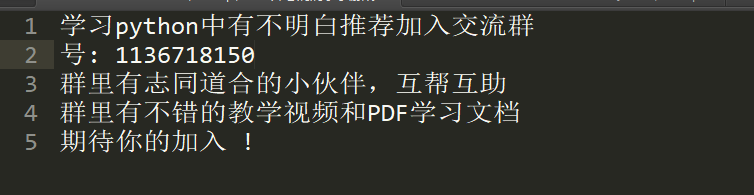数据探索
本文的数据集是经过清洗的美国某地区的房价数据集
import pandas as pd
import numpy as np
import seaborn as sns
import matplotlib.pyplot as plt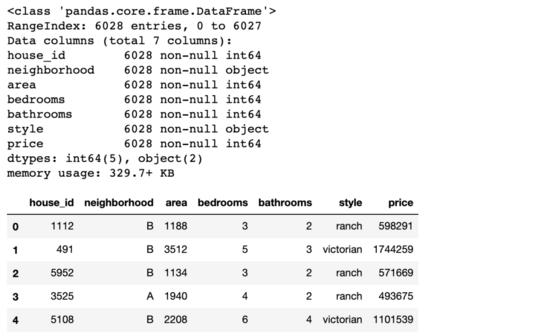参数说明：
neighborhood/area:所属街区和面积bedrooms/bathrooms:卧室和浴室style:房屋样式
多元线性回归建模
现在我们直接构建多元线性回归模型
from statsmodels.formula.api import ols
# 小写的 ols 函数才会自带截距项，OLS 则不会
# 固定格式：因变量 ~ 自变量(+ 号连接)
lm = ols('price ~ area + bedrooms + bathrooms', data=df).fit()
lm.summary()

红框为我们关注的结果值，其中截距项 Intercept 的 P 值没有意义，可以不用管它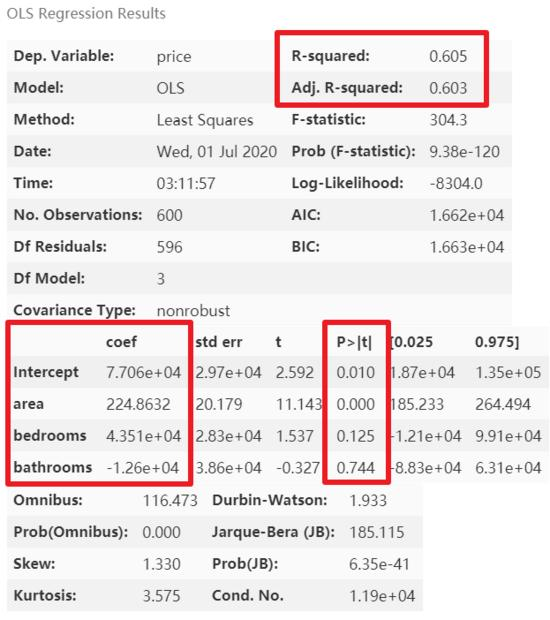模型优化
从上图可以看到，模型的精度较低，因为还有类别变量 neighborhood 和 style 没有完全利用。这里我们先查看一下类别变量的类别分布 情况：
# 类别变量，又称为名义变量，nominal variables
nominal_vars = ['neighborhood', 'style']

for each in nominal_vars:
print(each, ':')
print(df[each].agg(['value_counts']).T)  # Pandas 骚操作
# 直接 .value_counts().T 无法实现下面的效果
## 必须得 agg，而且里面的中括号 [] 也不能少
print('='*35)

虚拟变量的设置
因为类别变量无法直接放入模型，这里需要转换一下，而多元线性回归模型中类别变量的转换最常用的方法之一便是将其转化成虚拟变量。原理其实非常简单，将无法直接用于建模的名义变量转换成可放入模型的虚拟变量的核心就短短八个字：「四散拆开，非此即彼」。下面用一个只有 4 行的微型数据集辅以说明。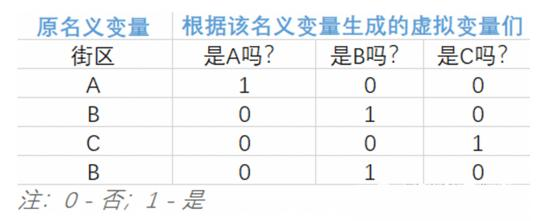从上表中，不难发现：
该名义变量有 n 类，就能拆分出 n 个虚拟变量巧妙的使用 0 和 1 来达到 「用虚拟变量列代替原名义变量所在类别」
接下来要做的就是将生成的虚拟变量们放入多元线性回归模型，但要注意的 是：「转化后的虚拟变量们需要舍弃一个」 ， 才能得到满秩矩阵。具体原因和有关线性代数的解释可以查看笔者打包好的论文，我们可以理解为，当该名义变量可划分为 n 类时，只需要 n-1 个虚拟变量就已足够获知所有信息了。该丢弃哪个，可根据实际情况来决定。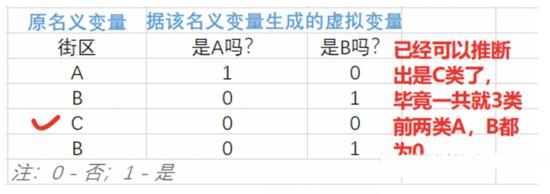因此为原数据集的某名义变 量添 加虚拟变量的步骤为:
抽出希望转换的名义变量(一个或多个)pandas 的 get_dummies 函数与原数据集横向拼接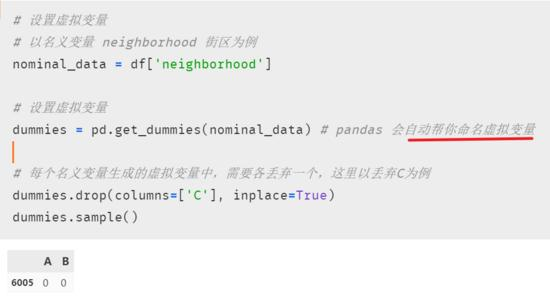注意虚拟变量设置成功后，需要与原来的数据集拼接，这样才能将其一起放进模型。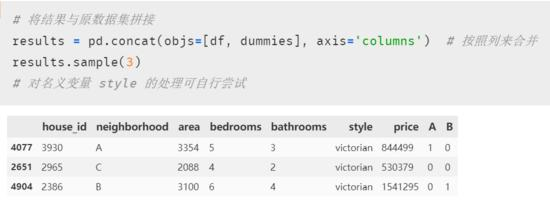再次建模后，发现模型精度大大提升，但潜在的多元共线性问题也随之显现出来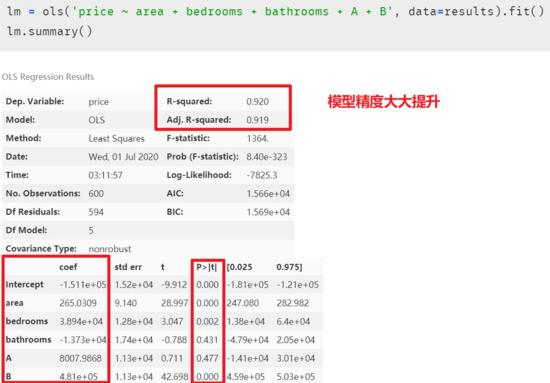在解释模型中虚拟变量的系数之前，我们先消除模型中多元共线性的影响，因为在排除共线性后，模型中的各个自变量的系数又会改变，最终的多元线性回归模型的等式又会不一样。多重线性回归模型的主要假设之一是我们的预测变量(自变量)彼此不相关。我们希望预测变量(自变量)与反应变量(因变量)相关，而不是彼此之间具有相关性。方差膨胀因子(Variance Inflation Factor，以下简称VIF)，是「指解释变量之间存在多重共线性时的方差与不存在多重共线性时的方差之比」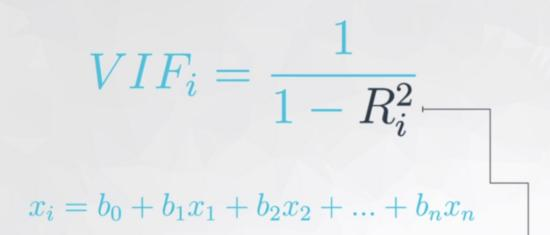上图公式可以看出在方差膨胀因子的检测中:
每个自变量都会有一个膨胀因子值，最后根据值的大小来选择是否删 减「既然表示相关性，是谁跟谁的相关性呢?」 是自变量中的某一变量与除它外剩余的自变量进行多元线性回归，取回归结果，即模型精度来作为这个变量与剩余自变量的相关性。听起来可能有点绕，这里举一下实例(用 “面积、卧室数量和浴室数量” 作为自变量来预测房价，在进行自变量的方差膨胀因子的检测时，面积、卧室数和浴室数轮流做单独的因变量，剩下的两个变量作为自变量，来看看这三个自变量中那个变量对其余两个变量的解释性高)越大，如已经到了 0.9，那分母就很小，的值就等于 10，即表示这个自变量已经同时解释了另外的某个或多个自变量，存在多元共线性，可以考虑删除一些自变量。
越大，显示共线性越严重。经验判断方法表明：「当 ，不存在多重共线性；当 ，存在较强的多重共线性；当 ，存在严重多重共线性」。
方差膨胀因子的检测
我们自己来写一个方差膨胀因子的检测函数
def vif(df, col_i):
"""
df: 整份数据
col_i：被检测的列名
"""
cols = list(df.columns)
cols.remove(col_i)
cols_noti = cols
formula = col_i + '~' + '+'.join(cols_noti)
r2 = ols(formula, df).fit().rsquared
return 1. / (1. - r2)

现在进行检测
test_data = results[['area', 'bedrooms', 'bathrooms', 'A', 'B']]
for i in test_data.columns:
print(i, '\t', vif(df=test_data, col_i=i))

发现 bedrooms 和 bathrooms 存在强相关性，可能这两个变量是解释同一个问题,方差膨胀因子较大的自变量通常是成对出现的。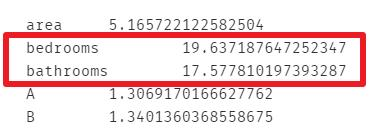果然， bedrooms 和 bathrooms 这两个变量的方差膨胀因子较高，这里删除自变量 bedrooms 再次进行建模
lm = ols(formula='price ~ area + bathrooms + A + B', data=results).fit()
lm.summary()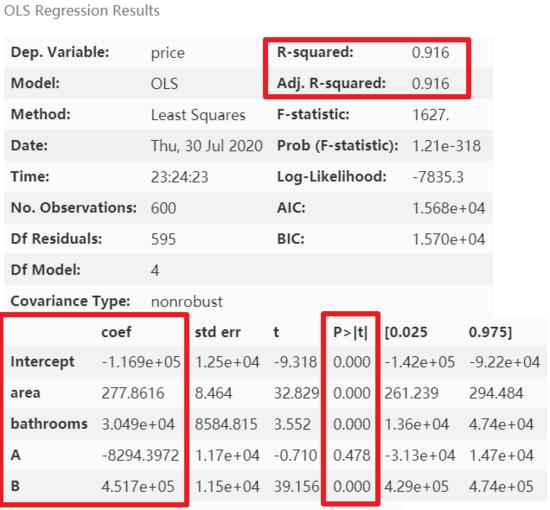模型精度稍降，但 消除了多元共线性后能够使模型的泛化能力提升 。再次进行多元共线性检测
test_data = results[['area', 'bedrooms', 'A', 'B']]
for i in test_data.columns:
print(i, '\t', vif(df=test_data, col_i=i))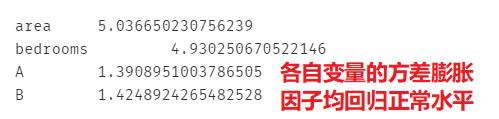那么多元共线性就 「只有通过方差膨胀因子才能看的出来吗？」 其实并不一定，通过 结合散点图或相关稀疏矩阵和模型中自变量的系数也能看出端倪 。下图是未处理多元共线性时的自变量系数。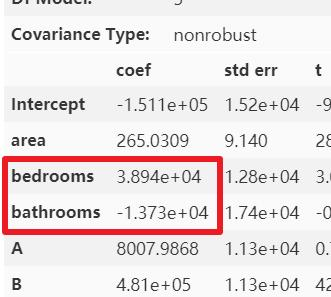可以很明显的看出， bathrooms 的参数很可能是有问题的，怎么可能 bathrooms 的数据量每增加一个，房屋总价还减少 1.373*10 的四次方美元呢？简单的画个散点图和热力图也应该知道房屋总价与 bathrooms 个数应该是成正比例关系的。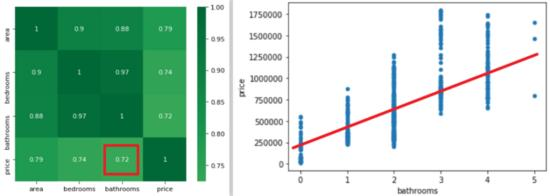模型解释
多元线性回归模型的可解释性比较强，将模型参数打印出来即可求出因变量与自变量的关系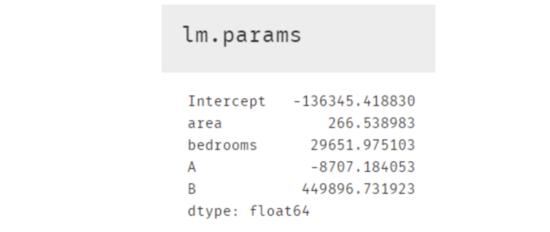所以最终的建模结果如下，且该模型的精度为 0.916
另外在等式结果中，截距项 Intercept 和 area , bedrooms 等变量的系数都还好理解；A，B 这两个 虚拟变量 可能相对困难些。其实根据原理部分的表格来看，如果房屋在 C 区，那等式中 A 和 B 这两个字母的值便是 0，所以这便引出了非常重要的一点： 使用了虚拟变量的多元线性回归模型结果中，存在于模型内的虚拟变量都是跟被删除掉的那个虚拟变量进行比较 。所以这个结果便表示在其他情况完全一样时(即除虚拟变量外的项)A 区的房屋比 C 区 低 8707.18 美元，B 区则比 C 区贵 449896.73.7 美元。当然我们也可以画个箱线图来查看与检验，发现结果正如模型中 A 与 B 的系数那般显示。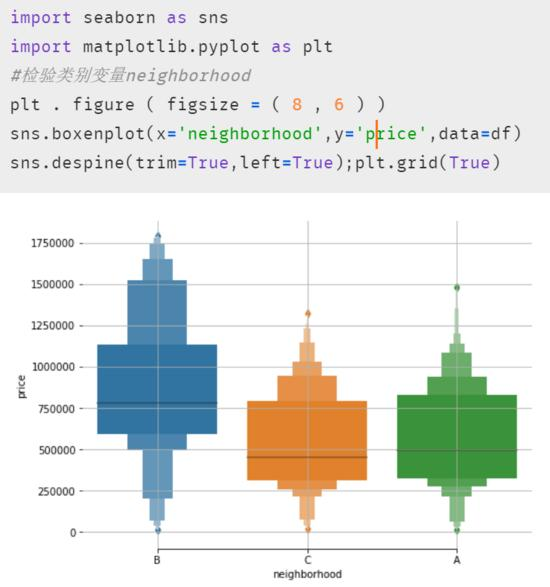结语： 本实践案例以多元线性回归为基础和前提，在因变量房价与多个自变量的实际观测值建立了多元线性回归模型；分析并检验各个预测变量对因变量的综合线性影响的显著性，并尽可能的消除多重共线性的影响，筛选出因变量有显著线性影响的自变量，对基准模型进行优化，并对各自变量相对重要性进行评定，进而提升了回归模型的预测精度。
展开全文...